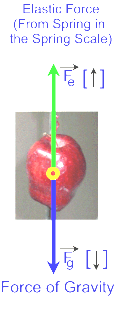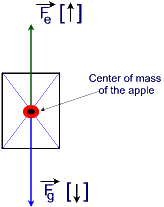#### Free Body Diagrams

A free body diagram (or sometimes abbreviated F.B.D.) is a simple sketch showing all the forces acting on an object when it is on its own (i.e. removed from its surrounding).  Here are some guidelines for drawing Free Body Diagrams.

When you draw a Free Body Diagram make sure that:

• Forces are represented by vectors
• A relative scale should be used to show the relative size of the forces whenever possible.
• Only the forces acting on the object should be included not the forces that the object exerts on other objects.
• All forces (vectors) should be drawn with respect to the center of gravity (or center of mass of the object).  This point is usually located at the geometric center of the object.

Example:  Consider the apple hanging from the spring scale below.To draw a free body diagram for the forces acting on the apple we would have to consider the apple alone, removed from the spring scale.Note the symbols used to represent the forces Fe is the elastic Force, Fg is the force of gravity.Note also the arrow head on top of the symbol indicating that the Force is a vector and finally note the direction of the forces; Fe is up while Fg is down.Finally note that the spring scale and the apple are not moving.  We say that they are in static equilibrium.  When this happens the forces on an object are said to be balanced.

Now consider the forces acting on apple removed from spring scale and drwa a free body diagram keeping in mind that:

• the elastic force Fe is pointing up
• the force of gravity Fg is pointing down